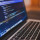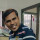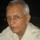1. Home >
2. Apps >
3. Groups >

# 5,8,13,?,34,55,89 Find the missing number

Question asked by SAURABH MISHRA in #Brainy Puzzles on Sep 2, 2019SAURABH MISHRA · Sep 2, 2019
Rank E2 - BEGINNER
Posted in: #Brainy PuzzlesAswini cse · Sep 2, 2019
Rank D1 - MASTER

Missing number is 21

5,8,13,?,34,55,89

5+8=13

34+55=89

so, 8+13=X

X = 21

In the given sequence,in order to get a number we need to add previous two numbers.

>> 5,8,13,21,34,55,89PIYUSH KUSHWAHA · Sep 5, 2019
Rank E1 - BEGINNER

@Aswini cse .. good job... Or you can subtract the previous two number to get your answer... Like 89-55=34, 55-34=21, 34-21=13, 21-13=8, 13-8=5.... :PParekh Priyanka · Sep 15, 2019
Rank E1 - BEGINNER

Missing number is 21Ramya Bhandaru · Feb 3, 2020
Rank D2 - MASTER

the missing number is 21

5 ,8 ,13 ,-----,34 ,55 ,89

5+8=13

13+x=34

34+55=89

x=34-13=21Venkat Sai Nakkina · Feb 3, 2020
Rank C3 - EXPERT

5,8,13,___,34,55,89

5+8=13

let me assume missing number is x

8+13=x

13+x=34

x=34-13=21

21 is the missing number.Ramani Aswath · Feb 3, 2020
Rank A1 - PRO

This is a part of the well known Fibanocci series: 0, 1,1,2,3,5,8,...............

The ratio of the last two numbers tend to the Golden Mean.